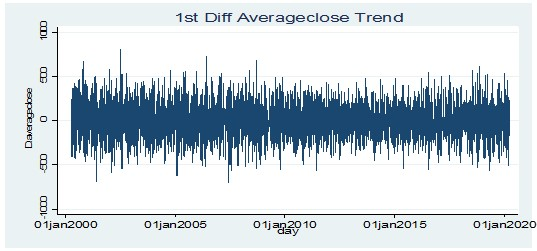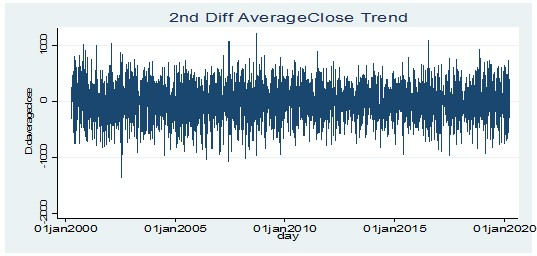# Forecasting growth stocks trend in the stock market with the ARIMA model

By Riya Jain & Priya Chetty on May 6, 2021

Investors in a financial market are empowered to plan their investment strategy to maximise their financial returns. For making sound investment decisions, different techniques are used to forecast the movement of stock prices. Among the technical analysis method of forecasting, the ARIMA model is considered a flexible statistical method of examining the behaviour of stocks and capturing the different types of data in one model (Mallikarjuna & Rao, 2019). However, stock price prediction is not only complex but also difficult (Adebiyi et al., 2014). This is due to dynamism, high volatility, complexity and uncertainty in the stock market. The existence of macro-economic factors like human behaviour and international events too influence the movement of the stock market.

This article examines the nature of one such type of stock, i.e. growth stock among 303 Bombay Stock Exchange-listed companies using the ARIMA model. Their future trend is then predicted. The period of data under consideration is 1st April 2000 to 31st March 2020. Stationary nature is assessed by Augmented Dickey-Fuller test, series correlation presence is determined by Correlogram, and partial correlation presence is administered by Partial Correlogram at 5% or 10% level of significance.

## Stationarity test for growth stocks for the ARIMA model

A time series is considered to be stationary if its statistical property doesn’t change with time. The presence of non-stationarity makes the predicted results spurious and unreliable. Thus it is essential to make the data stationary. The below sections examine the nature of growth stocks in case of average closing price, and average return.

### Average closing price

For the closing price, an assessment of the nature is shown in the table below.

The P-value of the average close price is 0.00 which is less than the significance value of 0.05 and 0.10. Thus, the null hypothesis of a unit root in the dataset is rejected. The below figure further verifies the statistical test results and depicts that the time variable does influence the nature of the average closing price.

Table 1 further examines the nature of the average closing price at 1st order difference wherein the p-value is 0.00 < 0.05 or 0.10. Moreover, the absolute test statistic value is greater than the critical significance value. Hence, the null hypothesis of a unit root in the dataset is rejected. These statistical results of the 1st order difference level can be verified by the below shows a figure which shows that there is no effect of time on the movement of the closing price.Figure 2: Stationarity test for average closing price at 1st order difference level for the ARIMA model

Even for the 2nd order difference level of average closing price, the p-value is 0.00 which is less than 0.05 or 0.10 and the absolute test statistic is greater than the critical significance value. Thus, the null hypothesis of unit root presence is rejected. The below figure also support the statistical result wherein, time doesn’t have an influence on stock closing price movement.Figure 3: Stationarity test for average closing price at 2nd order difference level for the ARIMA model

Thus, the average closing price is stationary at 0, 1st and 2nd order difference levels.

### Average return

Examination of the average return stationarity nature is shown in Table 2.

The above table depicts that the p-value is 0.00 which is less than 0.05 or 0.10 and even the absolute test statistic value is more than the absolute critical value. Thus, the null hypothesis of a unit root in the dataset is rejected. Figure 4 further supports the statistical results of average return wherein time doesn’t impact the movement of average return.

For the 1st order difference level too, the p-value is 0.00 < 0.05 or 0.10 and the test statistic absolute value is more than the critical absolute value. Thus, the null hypothesis of unit root presence in the dataset is rejected. Furthermore, the value of the statistical result is supported by the below figure wherein time doesn’t have much influence on the average return movement.

2nd order difference level for the average return p-value is 0.00 which is less than the required value of 0.05 or 0.10. Even the absolute test statistic value is higher than the absolute critical value. Thus, the null hypothesis of having unit root presence is rejected. Figure 6 supports the statistical results of the 2nd order difference value of average return wherein the time doesn’t have much impact on the movement on average return.

Hence, the stationary nature of the average return is derived at 0, 1st and 2nd difference levels.

## Correlogram test for growth stocks for the ARIMA model

A correlogram is a plot used for determining the presence of autocorrelation in the dataset. Herein, the examination of the serial correlation of current stock prices with their past values is shown below figure.

### The average closing price of growth stocks

In the above figure shaded region depicts the acceptance region for the moving average of the average close price while all the lines represent the different lags. As for all the lag levels, the lines are outside the acceptance region, thus, the optimal lag level cannot be derived at this level. However, the moving average could take value any value starting from 1. In order to derive the lag, the correlogram for the average closing price is examined at the 1st order differenced level.

The above figure shows that after the 1st lag all other autocorrelation value lies within the acceptance region, thus, the lag for the average closing price is 1, 22, and 36. Thus, 1, 22 and 36 is considered as the moving average for the closing price of growth stocks.

Furthermore, the assessment of the correlogram at the 2nd order difference level depicts that the autocorrelation value except for 1, 2, 35 or 36 lags within the acceptance region. Thus, the lag value for the average closing price at the 2nd order difference is 1, 2, 35 or 36 representing that the possible moving average could be 1, 2, 35 or 36.

### The average return of growth stocks

For the average return of stocks, the examination of the presence of serial correlation is shown in the figure below. Having all the values within the acceptance region except for the 1st lag, the lag level is determined. Thus, beyond lag level 1 there is no autocorrelation presence in the current return and the previous year’s returns. For forecasting growth stocks performance, the lag level for average return is 1. Hence, 1 is the moving average considered for the average return.

Furthermore, the assessment of average return at 1st order difference level shows that as except for lag at level 1, the values are in the acceptance region. Thus, the lag level for the 1st order differenced average return could be 1. Hence, the moving average at 1st order difference is 1.

Figure 12 below shows the average return correlogram analysis at the 2nd difference level. Herein, except for lags 1 and 2, the autocorrelation value is within the acceptance region. Thus, lag is derived at 1 and 2 showing that the moving average for the average return at the 2nd order difference level is 1 and 2.

## Partial correlogram test for growth stocks

The partial correlogram is a graphical analysis used for examining the presence of partial autocorrelation in a dataset. Herein, a partial correlogram is used to assess the relationship between the current value of stocks with their previous values.

### The average closing price of growth stocks

In the case of average closing price, the partial correlogram analysis shown in Figure 13 below depicts that other than 5 lags, the partial autocorrelation value is in the acceptance region. Hence, for the forecasting model, the autoregressive could be from 1 to 5.

Figure 14 below shows that for the first 4 lags, the value is beyond the acceptance region. Thus, in order to forecast the average closing price for the growth stocks, 1 to 4 is the lag for the average closing price. Hence, as partial correlation is present at a lag of 1 to 4, the autoregressive level considered for the forecasting model of average closing price could be from 1 to 4.

Examination of the partial correlogram at the 2nd order difference level depicts that the partial autocorrelation value is within the acceptance region except for the lag from 1 to 3. Thus, in order to build the forecasting model for the average closing price, the autoregressive could be from 1 to 3.

### The average return of growth stocks

The analysis of the partial correlogram for the average return in the case of growth stocks is shown in figure 16. Except for 1 and 5, the partial autocorrelation lies within the acceptance region. Thus, a forecasting model at 0 levels of difference could be formulated by having an autoregressive of 1 or 5.

At the 1st order difference level of average return, the partial autocorrelation value is outside the acceptance region for the lag value of 1 to 4. Thus, a forecast model for the average return could be built with autoregressive as 1 to 4.

Figure 18 depicts that the partial autocorrelation value is beyond the acceptance region for the lag value of 1 to 3. Thus, in order to build the average return forecasting model at the 2nd order difference level, the autoregressive could be from 1 to 3.